# Annotated heatmaps

```  # Generate random data
n <- 50; p <- 20
x <- abs(rmatrix(n, p, rnorm, mean=4, sd=1))
x[1:10, seq(1, 10, 2)] <- x[1:10, seq(1, 10, 2)] + 3
x[11:20, seq(2, 10, 2)] <- x[11:20, seq(2, 10, 2)] + 2
rownames(x) <- paste("ROW", 1:n)
colnames(x) <- paste("COL", 1:p)
## Scaling
aheatmap(x, scale = "row")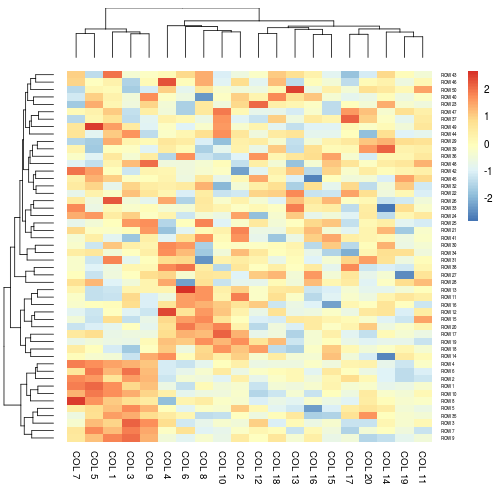aheatmap(x, scale = "col") # partially matched to 'column'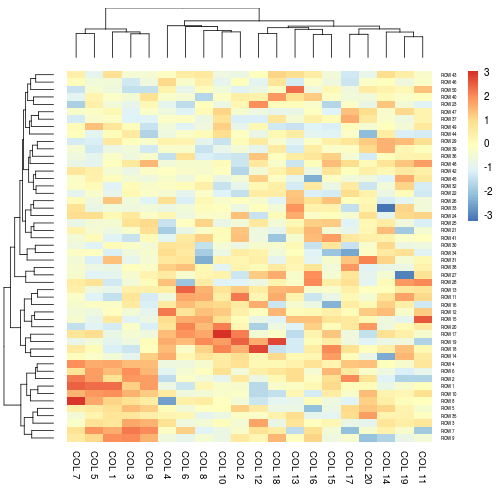aheatmap(x, scale = "r1") # each row sum up to 1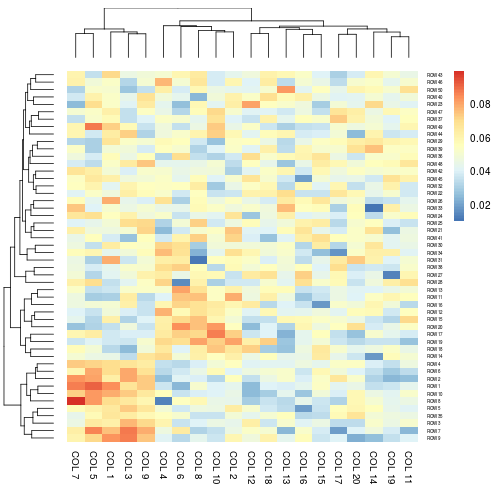aheatmap(x, scale = "c1") # each colum sum up to 1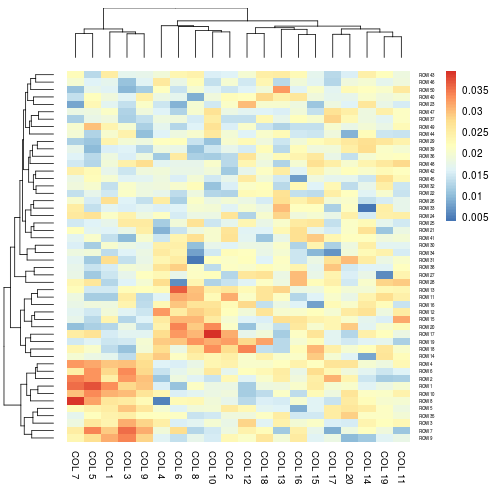## Heatmap colors
aheatmap(x, color = colorRampPalette(c("navy", "white", "firebrick3"))(50))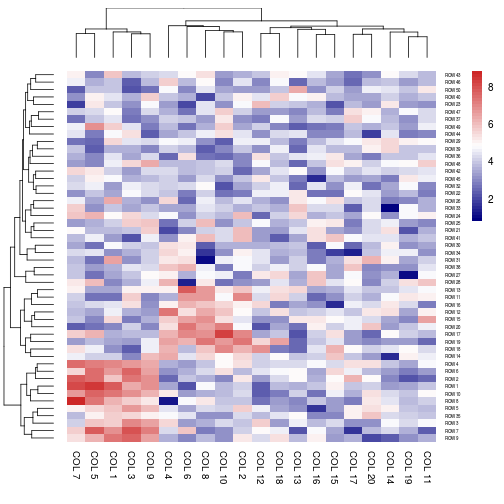# color specification as an integer: use R basic colors
aheatmap(x, color = 1L)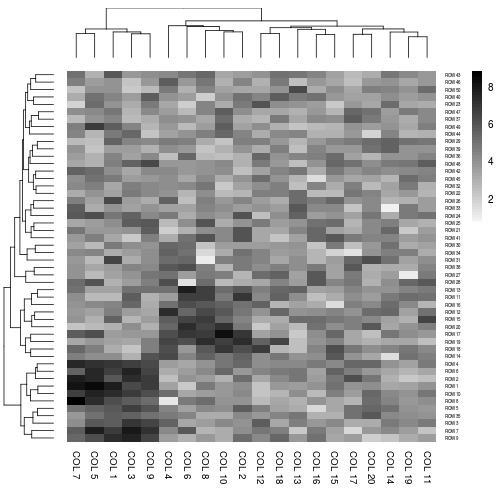# color specification as a negative integer: use reverse basic palette
aheatmap(x, color = -1L)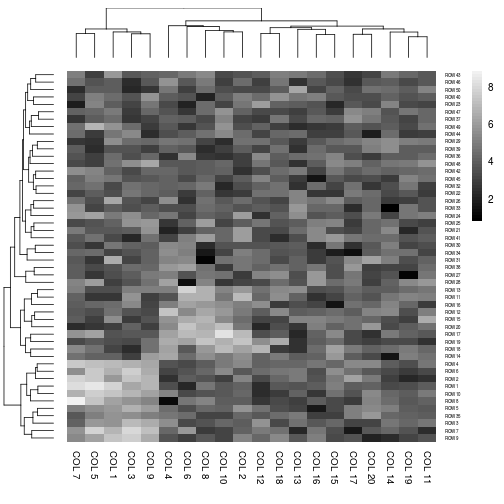# color specification as a numeric: use HCL color
aheatmap(x, color = 1)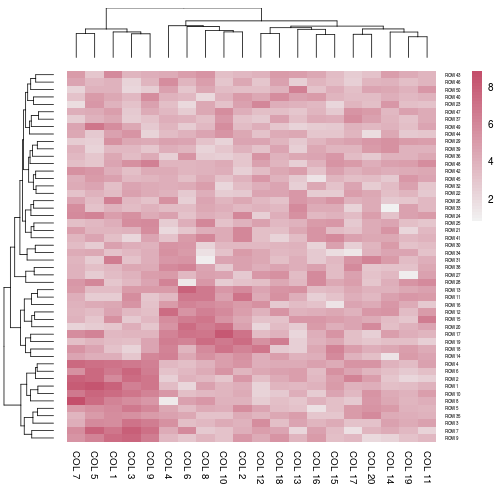# color for NA values
y <- x
y[sample(length(y), p)] <- NA
aheatmap(y)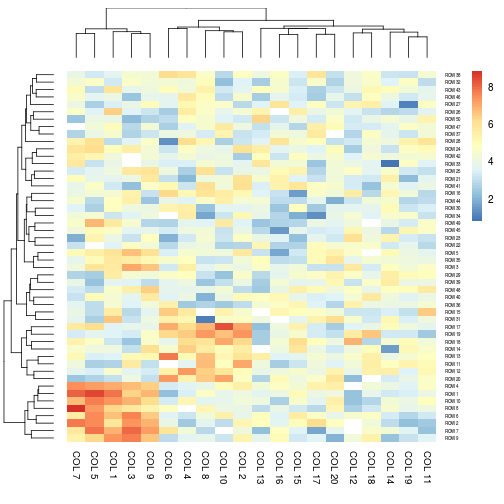aheatmap(y, na.color = 'black')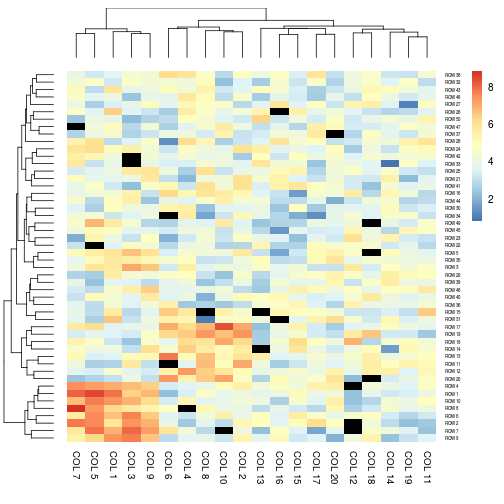# do not cluster the rows
aheatmap(x, Rowv = NA)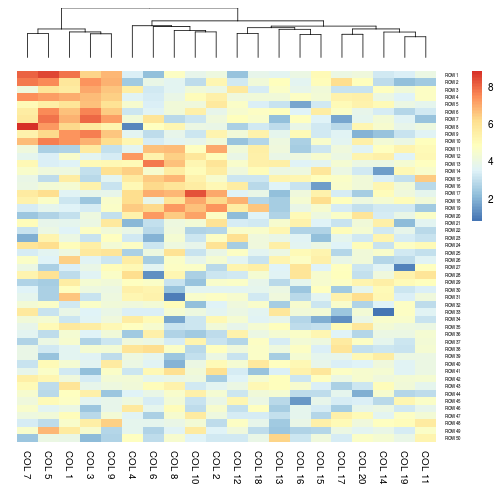# no heatmap legend
aheatmap(x, legend = FALSE)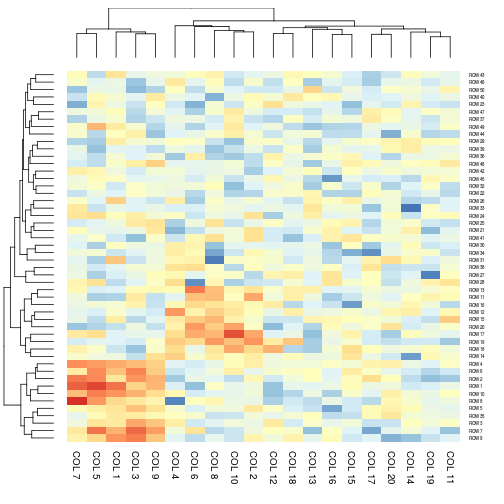# cell and font size
aheatmap(x, cellwidth = 10, cellheight = 5)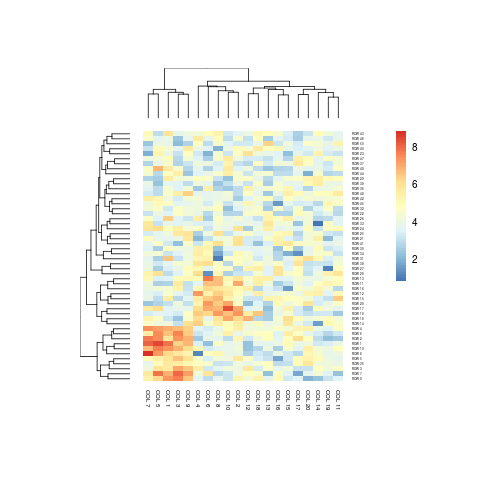# directly write into a file
aheatmap(x, cellwidth = 15, cellheight = 12, fontsize = 8, filename = "aheatmap.pdf")
# Generate column annotations
annotation = data.frame(Var1 = factor(1:p %% 2 == 0, labels = c("Class1", "Class2")), Var2 = 1:10)
aheatmap(x, annCol = annotation)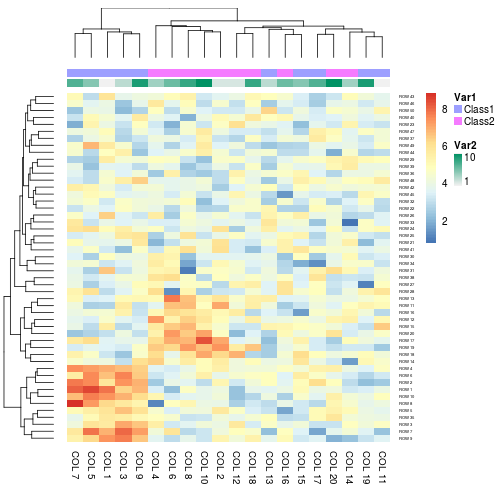aheatmap(x, annCol = annotation, annLegend = FALSE)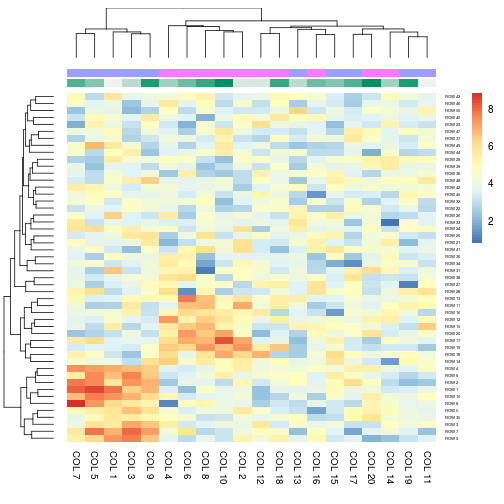# Specify colors
Var1 = c("navy", "darkgreen")
names(Var1) = c("Class1", "Class2")
Var2 = c("lightgreen", "navy")
ann_colors = list(Var1 = Var1, Var2 = Var2)
aheatmap(x, annCol = annotation, annColors = ann_colors)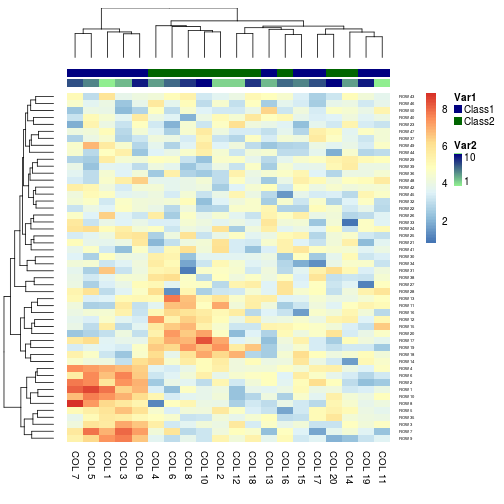# Specifying clustering from distance matrix
drows = dist(x, method = "minkowski")
dcols = dist(t(x), method = "minkowski")
aheatmap(x, Rowv = drows, Colv = dcols)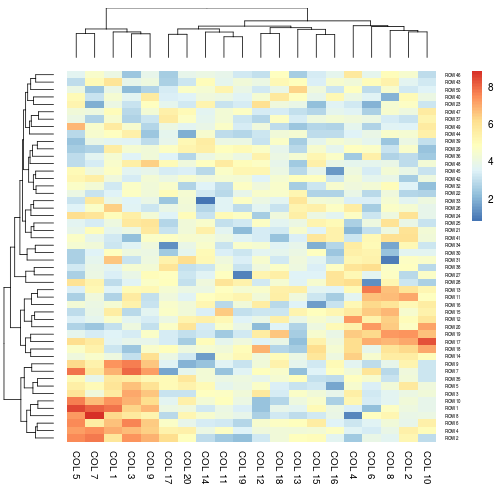# Display text in each cells
t <- outer(as.character(outer(letters, letters, paste0)), letters, paste0)[1:n, 1:p]
aheatmap(x, txt = t)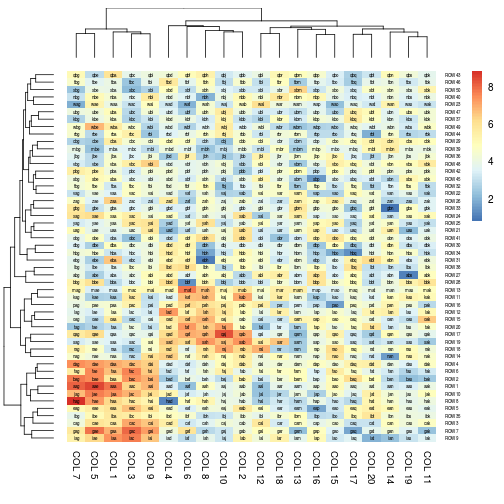# NA values are shown as empty cells
t.na <- t
t.na[sample(length(t.na), 500)] <- NA # half of the cells
aheatmap(x, txt = t.na)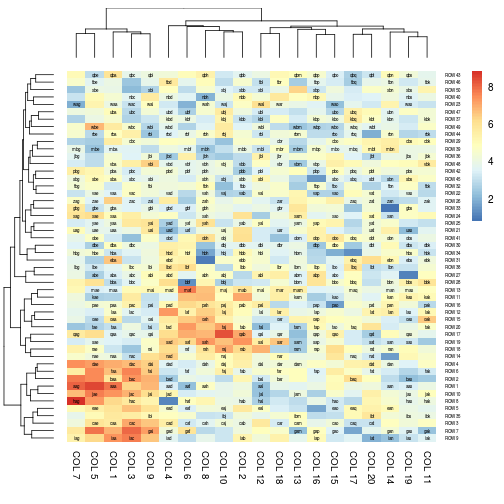# Borders
# all
aheatmap(x, annCol = annotation, border = TRUE)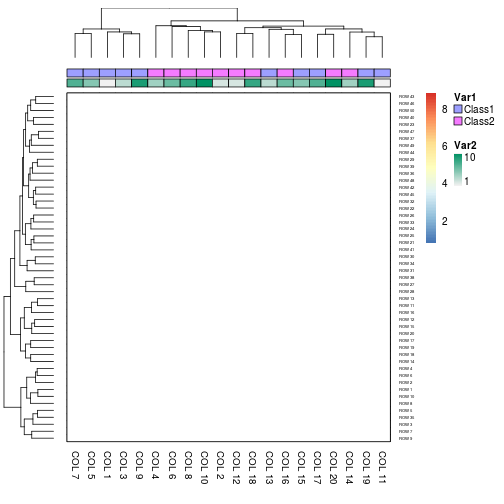# around data matrix
aheatmap(x, annCol = annotation, border = list(matrix = TRUE))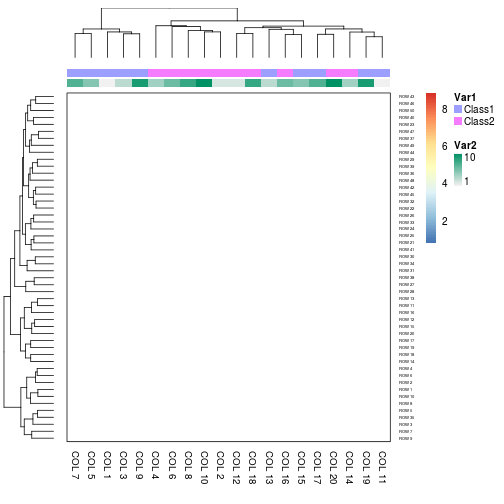# around cells only
aheatmap(x, annCol = annotation, border = list(cell = TRUE))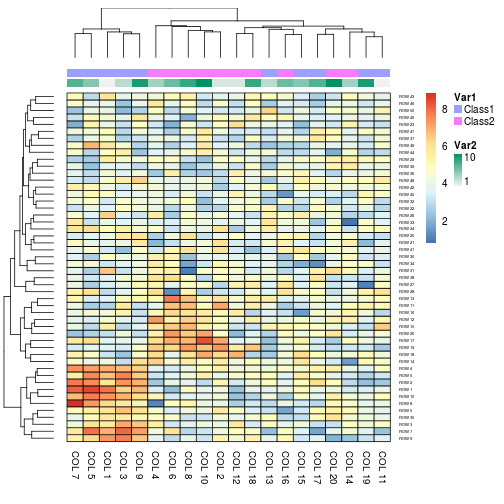# finer control
aheatmap(x, annCol = annotation, border = list(matrix = list(col = 'blue', lwd=2), annCol = 'green', annLeg = 'grey'))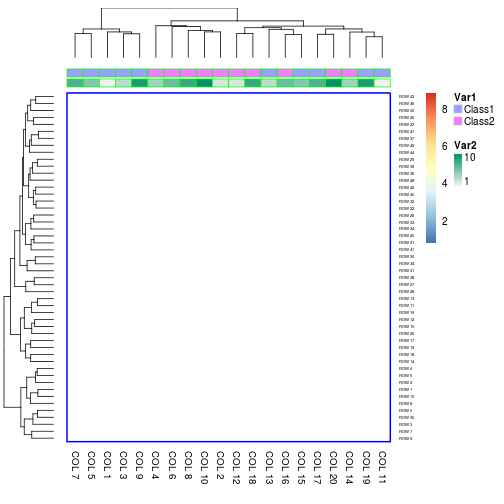```